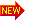# MATH BOARD GAMES FOR KIDS

These math board games are suitable for children and include: math croc board games, math coordinate board games, math footbal games, math zombie board games, math card games, math puzzle board games, math game puzzles, math maze board games and more. For kids, kindergarten, 1st grade, 2nd grade to 6th grade. The following topics are covered in the form of board games: addition, comparisons, counting numbers, fractions, mixed operations, telling time, division, multiplication, place value, powers, algebra, signs in math, ratios, spelling numbers, intergers, LCM & HCF etc. Download and print out these games as free PDF printables.

• ## Math GamesBoard Games - Interactive online: Pre-K & K | 1st Grade | 2nd Grade |3rd Grade | 4th Grade | 5th Grade | 6th Grade | 7th Grade |

## Math Card Games - PDF printable games

### 10) Comparison of numbers / inequalities - Greater than >, Less than <, Equal to =Download free PDF printable Math card games for kids here

### - Math powerpoint games with free templates

Cool math for kids. Math fun Exercises for kids, Fun Games, Printables board games, Quizzes, Printables, Puzzles- For Teachers, Parents, Educators and young learnersThere are free online exercises. Cool math for kids exercises will help kids practice Mathematics in a fun way. Kids relate to cool math games very well. We offer free games for kids like: Snakes and ladders math games, football math games, basketball games, en garde duel math game, memory math games, fling the teacher math games, interactive math quizzes, math puzzles & free math board games like: Croc puzzle math game, coordinate math game, card math game, zombie maze math games, snakes and ladders board math games. We cover fun mathematics game topics like:

Math games include among others : memory games, Walk the plank, Fling the Teacher, En Garde Duel, Basketball Game, Penalty Shoot, snakes and ladders math games, puzzles, board math games, and more.

Math Games, Board Games, PDF Prints & Interactive Math Fun Games for Children.These math games are from kindergarten math, elementary math, first grade math, preschool children, free online exercises, for first grade,second grade, third grade, fourth grade, fifth grade, sixth graders levels of maths. The following topics are covered among other math fun games:This website is designed around highly interactive and fun math games adapted for kids. Children will through this site, have the opportunity to learn math with fun through diverse math games like: snakes and ladders math games, basketball math games, puzzle math games, croc math borad games, fling the teacher math games, memory math games, powerpoint interactive math games, printable PDT math games and diverse online mathematics quiz activities for kids of divers grades like Pre-k, Kindergarten, 1st grade, 2nd grade, 3rd grade, 4th grade, 6th grade, 7th grade and more. These math games are designed around topics like : Addition, subtraction, Geometry, Comparison, Algebra, Shapes, Time, Fractions, Decimals, Sequence, Division, Metric system, Logarithms, ratios, probability, multiplication, telling time, number theories, ratios, Money, percentages, shapes, numeration, multiples, place value, graphs, exponents, square roots, intergers, inequalities, factoring, equations, radicals, polynomials, counting, data analysis, operations and more>>

These mathematics interactive math exercises are suitable for kids and young children and range from multiple choice math quizzes, gap fill quizzes, matching exercises, hotspot quizzes with graphics and more for interactive math practice. These are also made more interesting through coolmath fun games.

Website General Content: